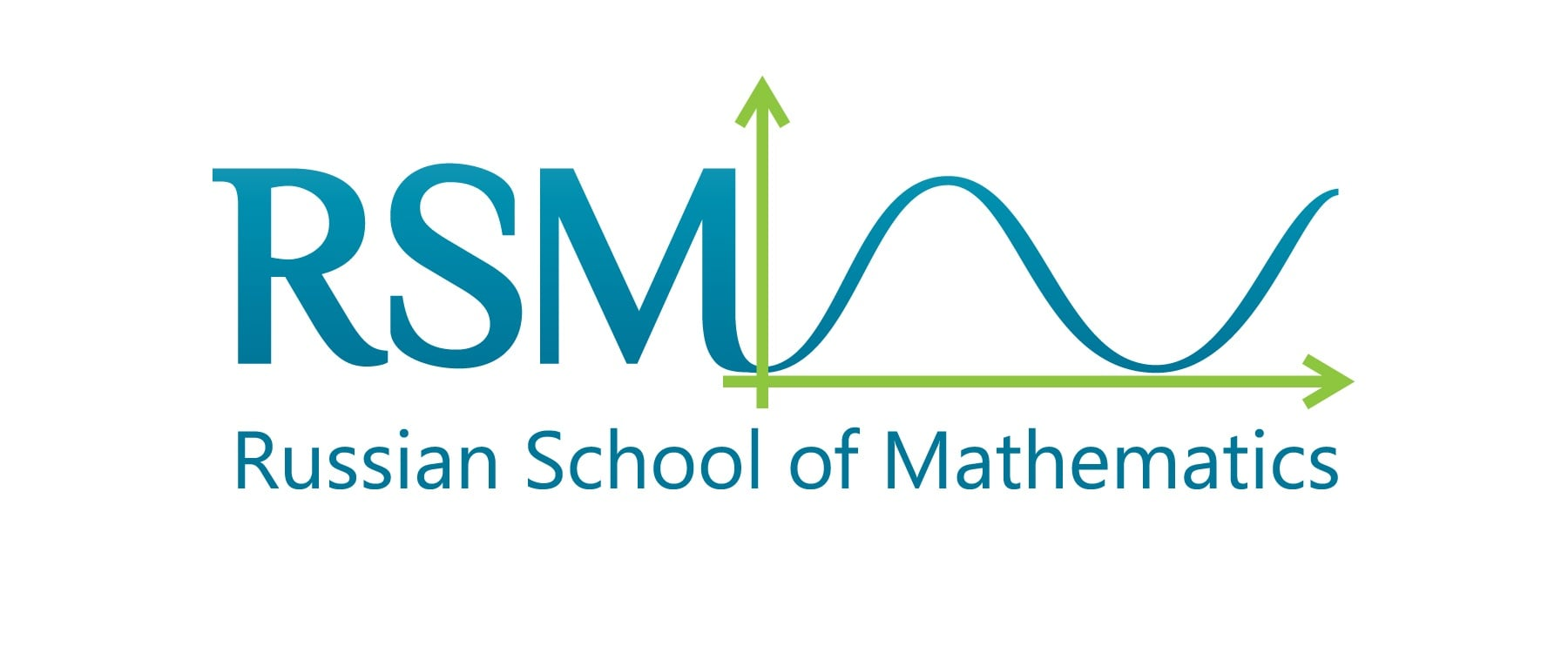What is better, a circle or a square? Happy Pi day!

It's Pi day today and everyone is preoccupied with problems about circles and circumference length. A circle, while appearing to be the simplest geometric shape, is also both fascinating and important. Let’s take a closer look at the incredible properties of a circle. What makes it so special? Is it, say, “better” than a square, and while we are on the subject - what is “better”, a cube or a sphere?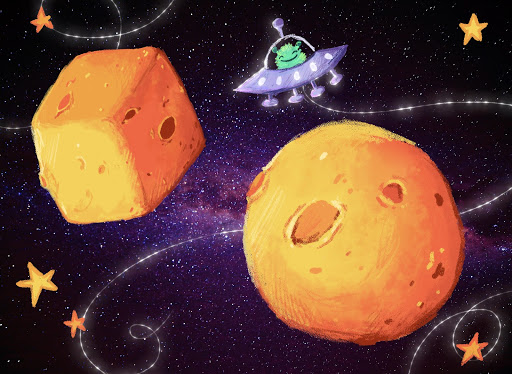Let’s start our dive into the world of circles with some food for thought.

Problem 1

Why are manhole covers made to be round, rather than square?

Solution.

Here are a few arguments in favor of the circle shape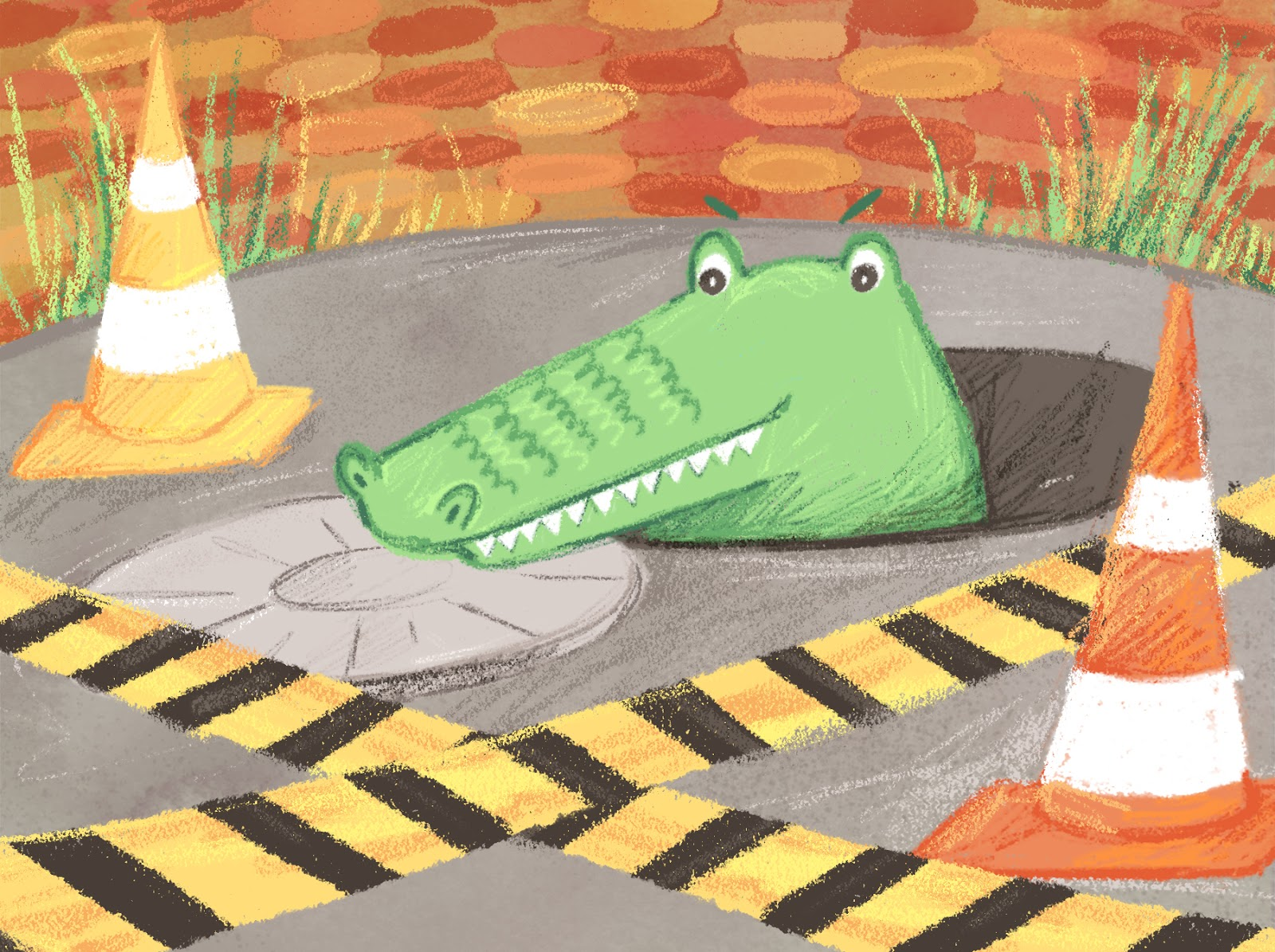• a round cover is easier to close, as one does not need to match the corners;

• one does not run the risk of having the square cover fall through the vent if it is not properly positioned. (A square cover poses this risk because the diagonal of a square is longer than its side. Hence, a vertically positioned square cover can fall through.)

• a round cover is easier to move: one can roll it on the ground like a wheel;

• a round cover takes up less area than a square of the same diameter, hence fewer materials are used in the manufacturing;

Perhaps, you will come up with even more arguments on your own. In this case, the circle is definitely better than the square!

Now, check out this surprise from the realm of science fiction showing an unbelievable reconciliation between what is circular and what is square.

Problem 2.

A space probe has landed on an asteroid. The only information we know is that the asteroid is both a sphere and a cube. The probe has moved along the surface to reach a point that is symmetrical to its starting point with respect to the center of the asteroid. During all of this time, the probe was continuously transmitting its coordinates to the space station where a 3D model of the probe’s movement trajectory was created. Is it possible that this information is insufficient to determine whether the probe was moving along a cube or a sphere?

Hint. We might want to say that while moving along the surface of a cube we will move from one side to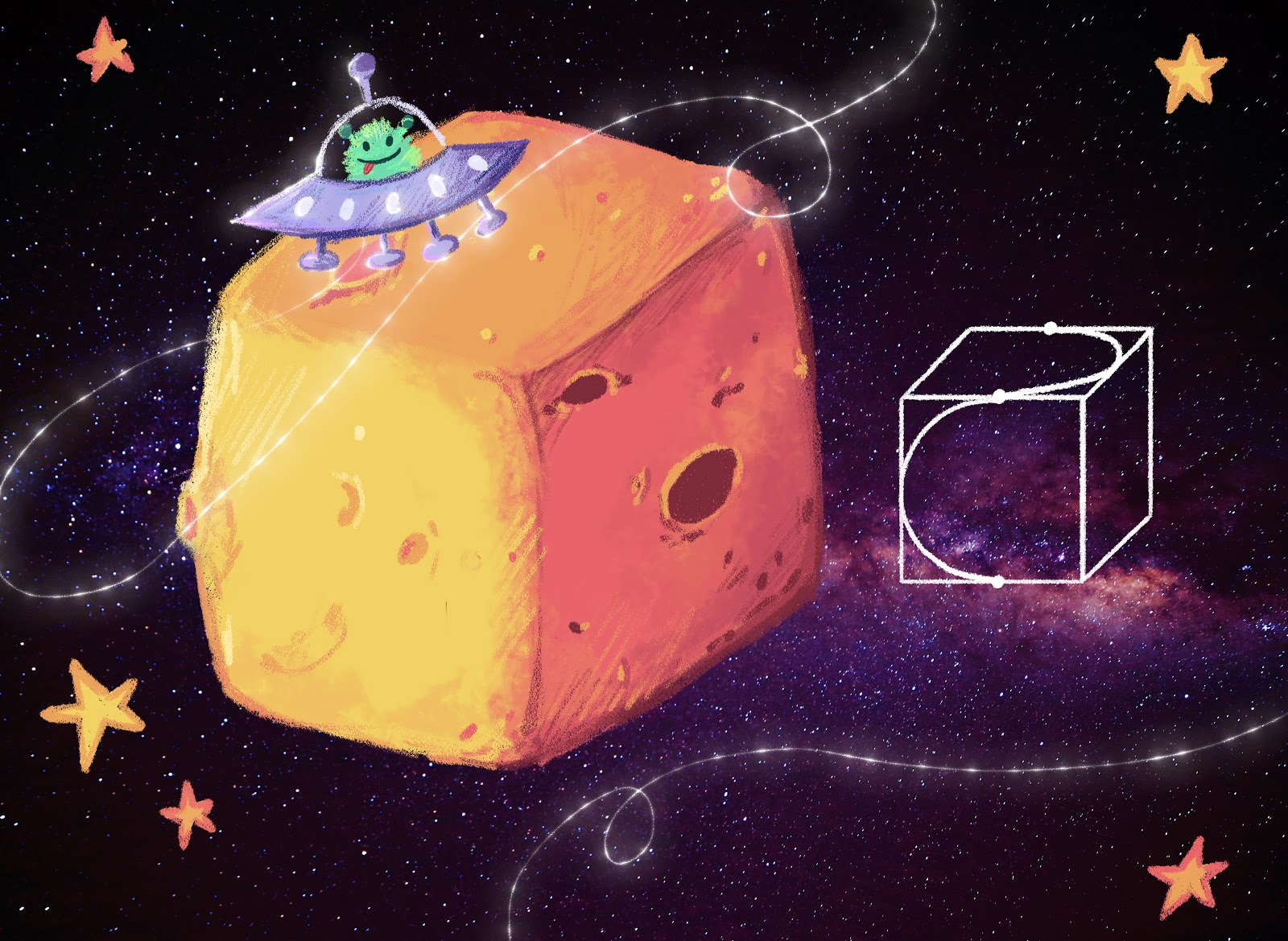another, that a curve will have a sharp turn. A sphere, on the other hand, is completely smooth. On the whole, who would not distinguish a cube from a sphere?

Let’s be systematic and to the point:

--- the center of the asteroid is calculated as the middle of the segment connecting the end points of the probes’ trajectory;

--- if the probe is moving along the cube in such a way that we are uncertain whether it might not be moving along the sphere with the same center, then this definitely shows that all of the points along the trajectory are at the same distance from the center of the cube.

 Hint. We might want to say that while moving along the surface of a cube we will move from one side to another, that a curve will have a sharp turn. A sphere, on the other hand, is completely smooth. On the whole, who would not distinguish a cube from a sphere?   Let’s be systematic and to the point:  --- the center of the asteroid is calculated as the middle of the segment connecting the end points of the probes’ trajectory; --- if the probe is moving along the cube in such a way that we are uncertain whether it might not be moving along the sphere with the same center, then this definitely shows that all of the points along the trajectory are at the same distance from the center of the cube. We have to ask ourselves the following questions: ---Consider point A on the cube’s surface. What other points on the cube’s surface are at the same distance from its center as point A? ---Is it possible, while moving along these points, to pass from one side of the cube to another side while gaining some ground distance, in general? The answers to these questions will guide you to the solution.   Solution. It is possible. Here is an example of a trajectory, consisting of two semicircles, that lies simultaneously on both the cube and the sphere.

Here are some fun hands on exercises. The great thing about a circle is that it’s like a Phoenix! You can cut it up in pieces, mix those up and then create a new circle! Now, don’t peek into our solutions. Pick up your scissors and, at first, try to do this on your own.

Problem 3.

A dot is placed somewhere inside a circle. Cut the circle into several parts and put those parts together to create a circle with the dot as its center.

Solution.

We can easily do it with three parts! Let O be the center of a given circle S, and A be the dot. When A and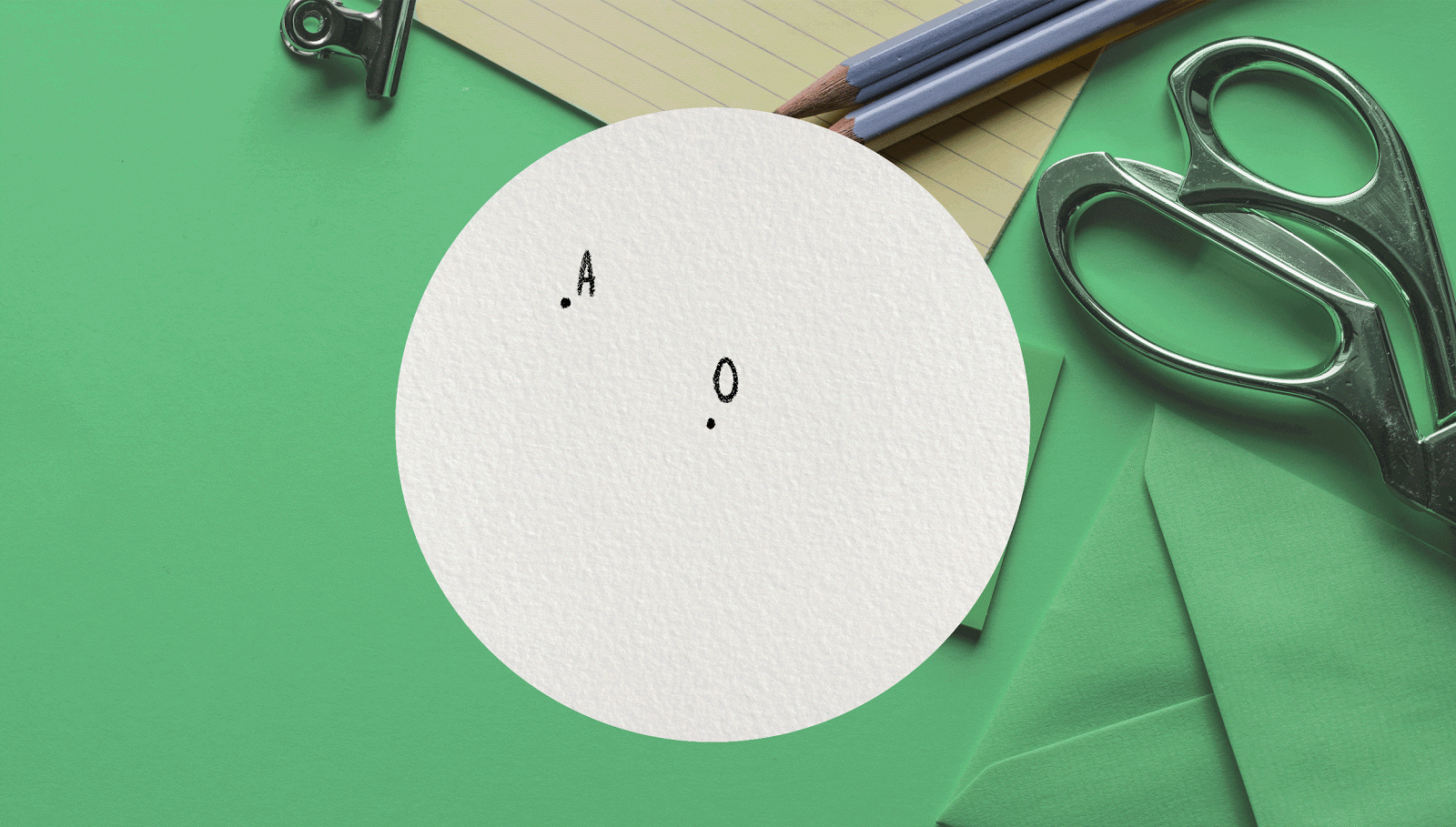O are in the same place, then no cutting is required. Otherwise, let’s cut out of the big circle S two identical small circles with A and O as their center and then switch these circles around. We are now left with a circle that has dot A as its center. As a result, we have three pieces of paper: two small circles and the rest is what remains of the big circle S after the little circles are removed.

How far can we take this? Is it possible to do this task by cutting the circle into two, rather than three, pieces? This looks a bit more challenging.

Problem 4.

A dot is placed somewhere inside a circle. Cut the circle into two parts to create a new circle with the dot as its center.

Hint. Let’s consider that the cut will form part of the edge of the new circle. In this case, the cut should follow the arc of the circle circumference with the dot at the center

Solution 1.

Let O be the center of a given circle S, and A be the dot. When A and O are in the same place, then no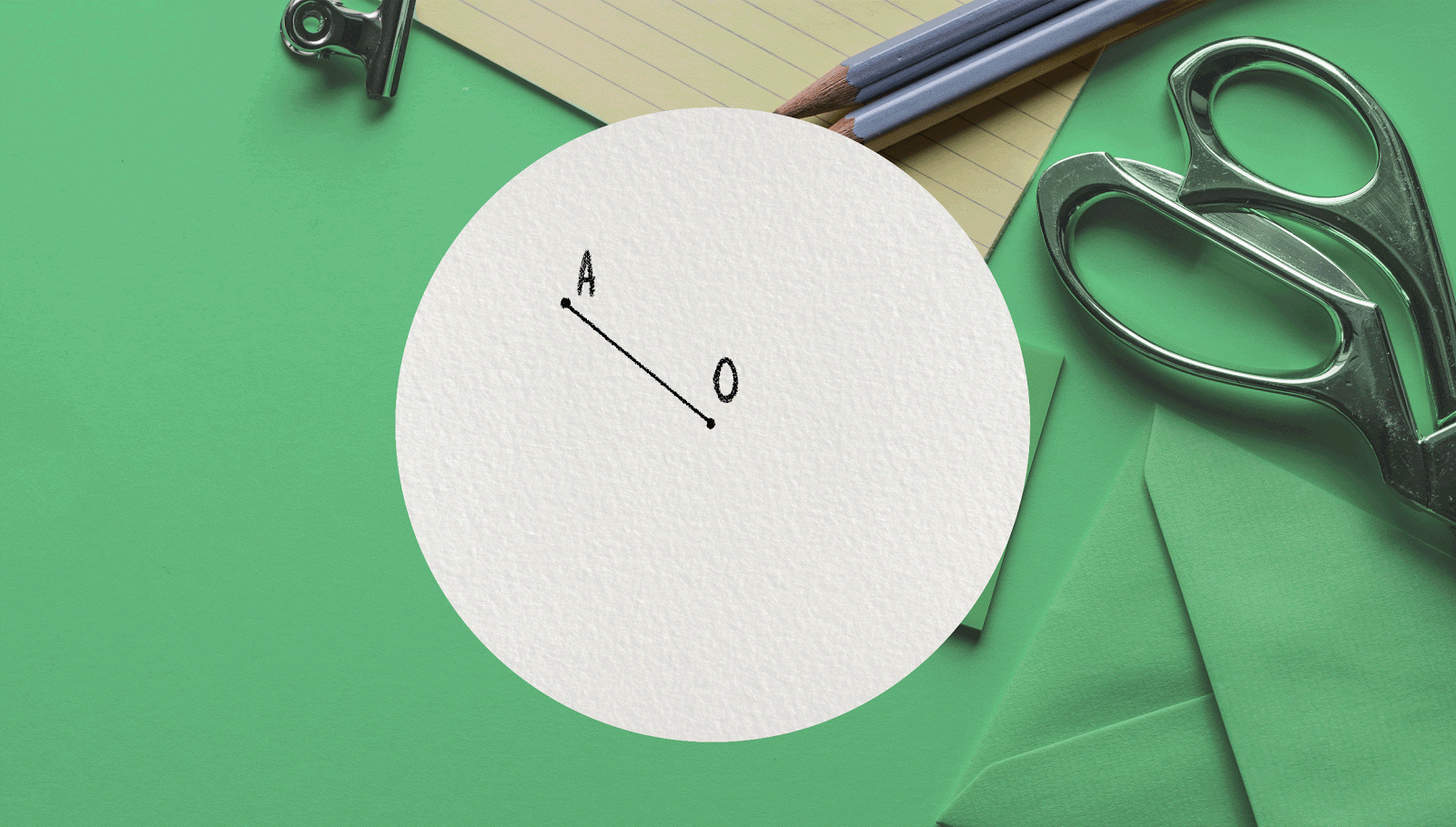cutting is required. Otherwise, let’s draw a circle S’ with center point A and the same radius as that of circle S. This new circle will split circle S into two parts. The first piece contains points A and O; it has an axis of symmetry m. We will leave this piece in its original place. We will position the second piece (in the shape of a crescent) to be symmetrical to its original place with respect to the axis m. Having done this, we find that both pieces together make up circle S’. The required task has been completed.

But there is  another solution to this problem! Don’t look, try to find it on your own first!

Solution 2.

Let’s cut along the circumference of a circle with the center in the middle of the segment OA and that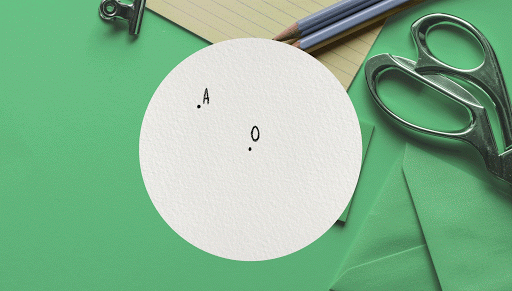completely falls within the given circle S. The result is two pieces. One is the new circle, the other piece is the remaining asymmetrical ring. Now, we turn the inner circle around its center until point A is in the same position with the center of circle S (point O).

So what’s the big deal? Perhaps there is nothing special about the circle? Maybe all of this can be done in the case of a square shape since a square also has a center.  Sounds easy, let’s give it a try!

Problem 5.

Replace the circle with a square in Problems 1 and 2. Solve these problems for the square shape.

Hint. The solution to problem 1 and the first solution to problem 2 apply almost exactly in this case. Ifdesired, one can change the second solution to problem 2 (replacing the inner circle with a rectangle) to apply to a square shape.

Amazingly, it turns out that the first two problems can be solved for either a circle or a square. However, the next problem shows the extent to which a circle shape can be more interesting and complex than a square. Take a look at the next problem. It would have been simple to solve for a square – we could have, for instance, cut it into 9 identical little squares. But we cannot solve the problem by cutting up a circle into 9 identical smaller circles!

Problem 6.

Cut up a circle into several equal parts so that at least one of the boundaries do not contain the center point of the circle.

Solution.

Let’s divide the circle with the center at point O into six equal parts with points A, B, C, D, E, and F. The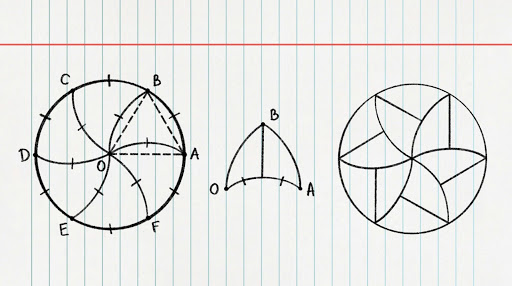result is the isosceles triangles OAB, OBC, OCD, ODE, OEF, OFA with sides OA = OB = OC = OD = OE = OF. Because the circle is divided into equal parts, the angle at point O for each of these triangles equals to 360 ÷ 6 = 60 degrees. For this reason, all of the triangles are equilateral. Let’s draw an arc of the circle with the center A and radius AB connecting point B to point O. Similarly, let’s draw arcs of circles with centers B, C, D, E, and F (see diagram).

In this manner, we have divided the circle into 6 equal parts. Each part has an axis of symmetry and therefore can be broken into two more equal parts. As a result, the circle is divided into 12 equal parts with its center being adjacent only to the six of these parts.   And we are not done, yet. Find other ways to solve this problem with the help of the diagrams.

The next argument in favor of the circle over the square is borrowed from real life. After all, all the reasons above were very abstract; what if it’s different in our day-to-day experience?

Tags: math,